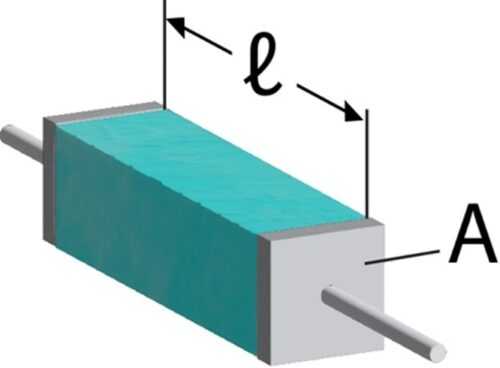# Understanding Resistivity & the 4 point probe Method

Conductive materials like adhesives, coatings, and greases are very useful in manufacturing, owing to their ease-of-use, cost-effectiveness, and performance. It is important however, to understand properties like resistivity (often referred to as volume resistivity, denoted ρ), so proper comparisons can be made between two materials. Unlike measurements for surface resistance which will vary with material thickness, resistivity is an intrinsic property and is therefore a better way to compare how conductive one material is relative to another. This paper describes resistivity as well as the 4 point probe method used to measure this property.

## Understanding Resistivity

Electrical conductivity is a measure of how well a material can carry an electrical charge. It is inversely proportional to resistivity and can be stated mathematically as σ = 1/ρ. Rather than report conductivity, it is conventional to report a material’s resistivity to gauge how conductive the material is. To help illustrate the concept of resistivity, imagine a conductive material as a rectangular prism of length l with cross-sectional area A. If we sandwich this material between 2 electrical contacts, we can measure the resistance across the length of material and calculate the resistivity. It is conventional to use centimeters for all dimensional measurements in this calculation. The equation is

ρ = R * A/l   this gives us units Ω cm

A graphical depiction of resistivity is shown below in figure 1.Figure 1: Depiction of a conductive material of length l and surface area A sandwiched between contacts

## The 4 Point Probe Method

Knowing how to measure resistivity is different than knowing how to measure resistance as you not only have to know how to measure micro ohm resistance but also prepare samples properly. The 4 point probe method is a relatively simple and robust method that allows you to measure the resistivity of thin films like our conductive coatings and conductive adhesives. Here, 4 parallel pins are lined up with 2 inner pins connected to a milli-ohmmeter and 2 outer pins connected to a current source. Samples are prepared by coating thin strips (approximately 4 inches long, 0.1 inches wide) onto a glass coupon. The probe is place onto the strip, a current is applied, and the resistance is measured by the milli-ohmmeter. By measuring the coating width and thickness, the surface area of the strip is calculated while the length is the distance between the 2 inner probes. We can then plug these values into our equation for resistivity to calculate a value.